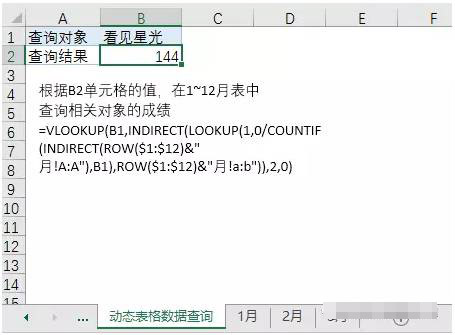# Excel 各种条件查询函数 单条件 多条件 精确 模糊 多结果

1,单条件单结果查询

VLOOKUP函数的语法非常简单。

=VLOOKUP(找谁，在哪里找，查找值在查找范围中的第几列？0失误找还是随便找？)

1，  查找值必须在查找范围的首列。

2，  第3参数是返回查找值在查找范围中的第几列，而不是表格的第几列。

3，  第4个参数是匹配方式，当使用精确匹配方式时，通常输入0。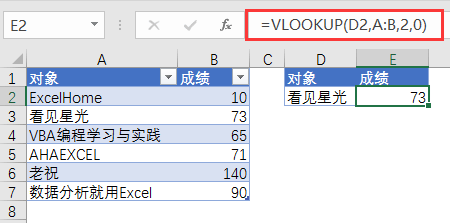=INDEX(A:A,MATCH(D2,B:B,0))

MATCH函数先取得D2单元格的值在B列中的序列号，然后通过INDEX函数按图索骥取得结果。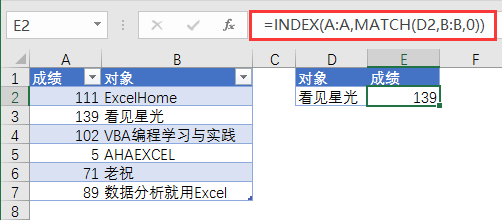{=VLOOKUP(D2,IF({1,0},B:B,A:A),2,0)}

2,多条件单结果查询

=LOOKUP(1,0/(条件1*条件2……),结果区域)

=LOOKUP(1,0/((A\$2:A\$7=E2)*(B\$2:B\$7=F2)),C\$2:C\$7)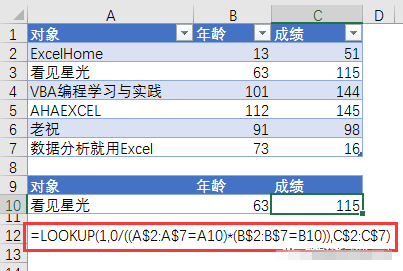3,单条件模糊查询

Excel内常用的通配符有两个。一个星号（*），一个问号（？）。

MATCH函数和VLOOKUP函数都支持通配符的使用。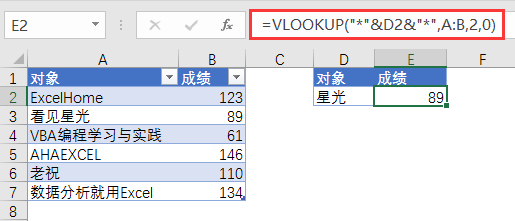4,多条件模糊查询

=LOOKUP(1,0/SEARCH("*"&A10&"*"&B10&"*",A\$2:A\$7&B\$2:B\$7),C\$2:C\$7)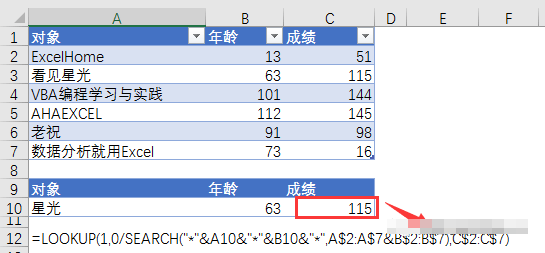5,多结果数据查询：

=INDEX(\$B:\$B,SMALL(IF(\$A\$1:\$A\$7=\$D\$2,ROW(\$1:\$7)),ROW(A1)))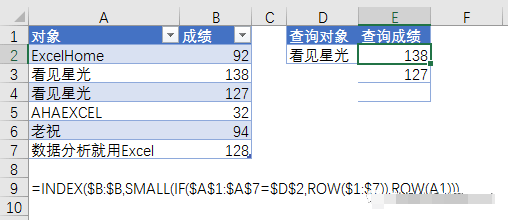6,动态表格数据查询

=VLOOKUP(B2,INDIRECT(B1&"!A:B"),2,0)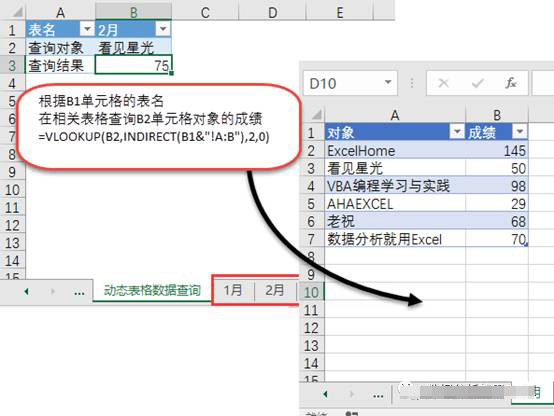7,多表格数据查询

=VLOOKUP(B1,INDIRECT(LOOKUP(1,0/COUNTIF(INDIRECT(ROW(\$1:\$12)&"月!A:A"),B1),ROW(\$1:\$12)&"月!a:b")),2,0)